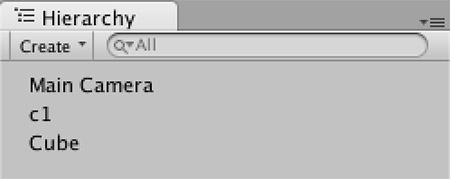# Jim's GameDev Blog# 矩阵基础 2

2016-5-16

### Case4``````// 使用三根基轴构建矩阵
// 如果只是用来变换法线的话，matR就已经够了，直接做变换操作即可
Matrix4x4 matR = Matrix4x4.identity;
matR.SetRow(0, new Vector4(c1.right.x, c1.right.y, c1.right.z, 0));
matR.SetRow(1, new Vector4(c1.up.x, c1.up.y, c1.up.z, 0));
matR.SetRow(2, new Vector4(c1.forward.x, c1.forward.y, c1.forward.z, 0));
newNormal = matR * new Vector4(normal.x, normal.y, normal.z, 0);

// 位移矩阵
Matrix4x4 matT = Matrix4x4.identity;
matT.SetRow(0, new Vector4(1, 0, 0, -c1.position.x));
matT.SetRow(1, new Vector4(0, 1, 0, -c1.position.y));
matT.SetRow(2, new Vector4(0, 0, 1, -c1.position.z));
// 缩放矩阵
Matrix4x4 matS = Matrix4x4.identity;
matS.SetRow(0, new Vector4(c1.localScale.x, 0, 0, 0));
matS.SetRow(1, new Vector4(0, c1.localScale.y, 0, 0));
matS.SetRow(2, new Vector4(0, 0, c1.localScale.z, 0));
// 如果用来变换顶点，那么需要同时使用 matR matT matS 这三个矩阵
// 注意相乘的顺序是非常重要的，不可颠倒
Matrix4x4 mat = matS * matR * matT;
newPos = mat * new Vector4(pos.x, pos.y, pos.z, 1);
``````